STRONG AND WEAK BASES

```
```

This page explains the terms strong and weak as applied to bases. As a part of this it defines and explains Kb and pKb.

We are going to use the Bronsted-Lowry definition of a base as a substance which accepts hydrogen ions (protons).

Note:  If you don't know what the Bronsted-Lowry theory is, you should read about theories of acids and bases on another page in this section. You don't need to spend time reading about Lewis acids and bases for the purposes of this present page.

The usual way of comparing the strengths of bases is to see how readily they produce hydroxide ions in solution. This may be because they already contain hydroxide ions, or because they take hydrogen ions from water molecules to produce hydroxide ions.

```
```

Strong bases

Explaining the term "strong base"

A strong base is something like sodium hydroxide or potassium hydroxide which is fully ionic. You can think of the compound as being 100% split up into metal ions and hydroxide ions in solution.

Each mole of sodium hydroxide dissolves to give a mole of hydroxide ions in solution.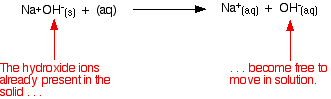Some strong bases like calcium hydroxide aren't very soluble in water. That doesn't matter - what does dissolve is still 100% ionised into calcium ions and hydroxide ions. Calcium hydroxide still counts as a strong base because of that 100% ionisation.

Working out the pH of a strong base

Remember that: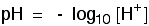Since pH is a measure of hydrogen ion concentration, how can a solution which contains hydroxide ions have a pH? To understand this, you need to know about the ionic product for water.

Important:  If you don't understand about the ionic product for water you must follow this link before you go on.

Wherever there is water, an equilibrium is set up. Using the simplified version of this equilibrium: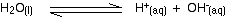In the presence of extra hydroxide ions from, say, sodium hydroxide, the equilibrium is still there, but the position of equilibrium has been shifted well to the left according to Le Chatelier's Principle.

Note:  If you don't understand Le Chatelier's Principle, you should follow this link before you go on. Make sure that you understand the effect of concentration on position of equilibrium.

There will be far fewer hydrogen ions than there are in pure water, but there will still be hydrogen ions present. The pH is a measure of the concentration of these.

An outline of the method of working out the pH of a strong base

• Work out the concentration of the hydroxide ions.

• Use Kw to work out the hydrogen ion concentration.

• Convert the hydrogen ion concentration to a pH.

An example

To find the pH of 0.500 mol dm-3 sodium hydroxide solution:

Because the sodium hydroxide is fully ionic, each mole of it gives that same number of moles of hydroxide ions in solution.[OH-] = 0.500 mol dm-3

Note:  You would have to be careful here if you had a base like calcium hydroxide, Ca(OH)2. Each mole of calcium hydroxide would produce twice as many hydroxide ions in solution.

Now you use the value of Kw at the temperature of your solution. You normally take this as 1.00 x 10-14 mol2 dm-6.[H+] [OH-] = 1.00 x 10-14

This is true whether the water is pure or not. In this case we have a value for the hydroxide ion concentration. Substituting that gives:[H+] x 0.500 = 1.00 x 10-14

If you solve that for [H+], and then convert it into pH, you get a pH of 13.7.

Note:  If you want more examples to look at and to try yourself (with fully worked solutions given), you may be interested in my chemistry calculations book. This also includes the reverse problem which is slightly more confusing - working out the concentration of a strong base from its pH.

Weak bases

Explaining the term "weak base"

Ammonia is a typical weak base. Ammonia itself obviously doesn't contain hydroxide ions, but it reacts with water to produce ammonium ions and hydroxide ions.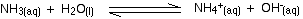However, the reaction is reversible, and at any one time about 99% of the ammonia is still present as ammonia molecules. Only about 1% has actually produced hydroxide ions.

A weak base is one which doesn't convert fully into hydroxide ions in solution.

```

```

Important:  What follows isn't required by any of the current UK A' level syllabuses.

Comparing the strengths of weak bases in solution: Kb

When a weak base reacts with water, the position of equilibrium varies from base to base. The further to the left it is, the weaker the base.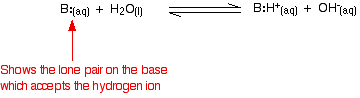You can get a measure of the position of an equilibrium by writing an equilibrium constant for the reaction. The lower the value for the constant, the more the equilibrium lies to the left.

In this case the equilibrium constant is called Kb. This is defined as: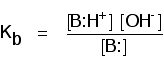Note:  If you want to know why the water has been omitted from the bottom of this expression, you will find it explained on the page about strong and weak acids under the corresponding constant, Ka.

pKb

The relationship between Kb and pKb is exactly the same as all the other "p" terms in this topic: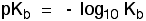The table shows some values for Kb and pKb for some weak bases.

baseKb (mol dm-3)pKb
C6H5NH24.17 x 10-109.38
NH31.78 x 10-54.75
CH3NH24.37 x 10-43.36
CH3CH2NH25.37 x 10-43.27

As you go down the table, the value of Kb is increasing. That means that the bases are getting stronger.

As Kb gets bigger, pKb gets smaller. The lower the value of pKb, the stronger the base.

This is exactly in line with the corresponding term for acids, pKa - the smaller the value, the stronger the acid.

Note:  If you are interested in exploring organic bases further, you will find them explained elsewhere on the site.

```
```
 Questions to test your understanding If this is the first set of questions you have done, please read the introductory page before you start. You will need to use the BACK BUTTON on your browser to come back here afterwards. questions on strong and weak bases answers
```
```

Where would you like to go now?

To the acid-base equilibria menu . . .

To the Physical Chemistry menu . . .

To Main Menu . . .

```
```

© Jim Clark 2002 (modified November 2013)